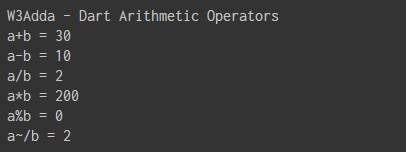# Dart Arithmetic operators

In this tutorial you will learn about the Dart Arithmetic operators and its application with practical example.

## Dart Arithmetic Operators

Arithmetic Operators are used to perform arithmetic operations like addition, subtraction, multiplication, division, %modulus, exponent, etc. Let variable a holds 20 and variable b holds 10, then −

Dart Arithmetic operators
Operator Name Description Example
`+` `Addition` ```Addition of given operands ``` `a+b returns 30`
`-` `Subtraction` `Subtraction of second operand from first` ```a-b returns 10 ```
`-expr` ```Unary Minus ``` `reverse the sign of the expression` ```-(a-b) returns -10 ```
`*` `Multiply` `Multiplication of given operands` ```a*b returns 200 ```
`/` `Division` ```Returns Quotient after division ``` ```a/b returns 2 ```
`~/` `Division(Int)` ```Return an integer result ``` ```a/b returns 2 ```
`%` `Modulus` `Returns Remainder after division` `a%b returns 0`

Example:-

When you run the above Dart program, you will see following output.

Output:-In this tutorial we have learn about the Dart Arithmetic operators and its application with practical example. I hope you will like this tutorial.